Algebra 1 : How to write expressions and equations

Example Questions

Example Question #131 : How To Write Expressions And Equations

Write the expression:  Sixteen less than a number squared.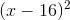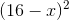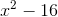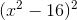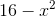Explanation:

Separate the sentence into parts.

A number squared:Sixteen less than a number squared:Note that the number 16 is subtract fromif 16 is less than the number.

The answer is:Example Question #132 : How To Write Expressions And Equations

Write as a variable expression:

Nine multiplied by the sum of a number and two thirds.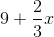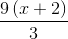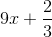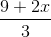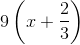Explanation:

"The sum of a number and two thirds" is the result of addingand: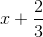Nine multiplied by this, remembering to use parentheses to override the precedence of multiplication, is:Example Question #133 : How To Write Expressions And Equations

Write as a variable expression:

Twenty added to the product of seven tenths and a number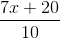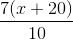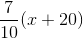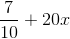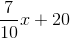Explanation:

"The product of seven tenths and a number" is the result of multiplying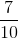and: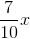.Example Question #134 : How To Write Expressions And Equations

Which of the following English-language expressions can be rewritten as the algebraic expression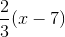?

The product of a number and seven, subtracted from two thirds

Two thirds multiplied by the difference of seven and a number

None of the other responses is correct

Two thirds multiplied by the difference of a number and seven

Two thirds subtracted from the product of a number and seven

Two thirds multiplied by the difference of a number and seven

Explanation:is two thirds multiplied by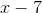, which is the difference of a number and seven. Putting this together, this expression is stated as:

"Two thirds multiplied by the difference of a number and seven"

Example Question #135 : How To Write Expressions And Equations

"If seven is multiplied by the sum of an unknown number and twelve, the result is four times that unknown number."

Rewrite the above statement as a variable equation.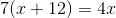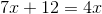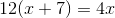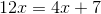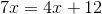Explanation:

If we call this unknown number, then "the sum of an unknown number and twelve" is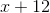"seven...multiplied by the sum of an unknown number and twelve" is seven multiplied by; remembering to override the order of operations with parentheses, the expression is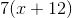The result is four times that unknown number, which is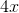Putting it together, the equation isExample Question #136 : How To Write Expressions And Equations

Write the expression:  One more than the quantity of twice a number squared.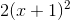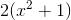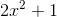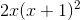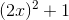Explanation:

Split the sentence into parts.  Let a variable be the number.

Twice a number:The quantity of twice a number squared: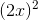One more than the quantity of twice a number squared:The answer is:Example Question #137 : How To Write Expressions And Equations

Write the expression:  Four less than half a number.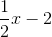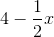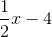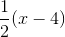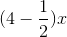Explanation:

Separate the sentence into parts.  Use a variable to denote the number.

Half a number: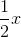Four less than half a number:The answer is:Example Question #138 : How To Write Expressions And Equations

Write the following equation:  Five more than twice a number is nine.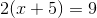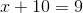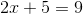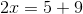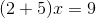Explanation:

Separate the sentence into parts.

Twice a number:Five more than twice a number: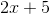Is nine: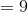Combine the parts.

The answer is:Example Question #139 : How To Write Expressions And Equations

Write the expression:  Six more than the cube root of twice a number.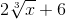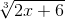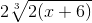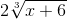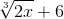Explanation:

Seperate the sentence into parts.

Twice a number:The cube root of twice a number: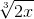Six more than the cube root of twice a number:The answer is:Example Question #140 : How To Write Expressions And Equations

"If the product of one-tenths and a number is subtracted from six, the result is seven more than that number."

Rewrite the above statement as a variable equation.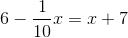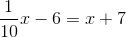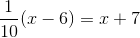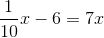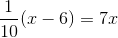Explanation:

If we call this unknown number, then "the product of one-tenths and a number" is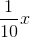.

"The product of one-tenths and a number...subtracted from six" issubtracted from six, or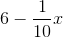.

This result is equal to seven more than that number, which is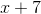, so the equation is.